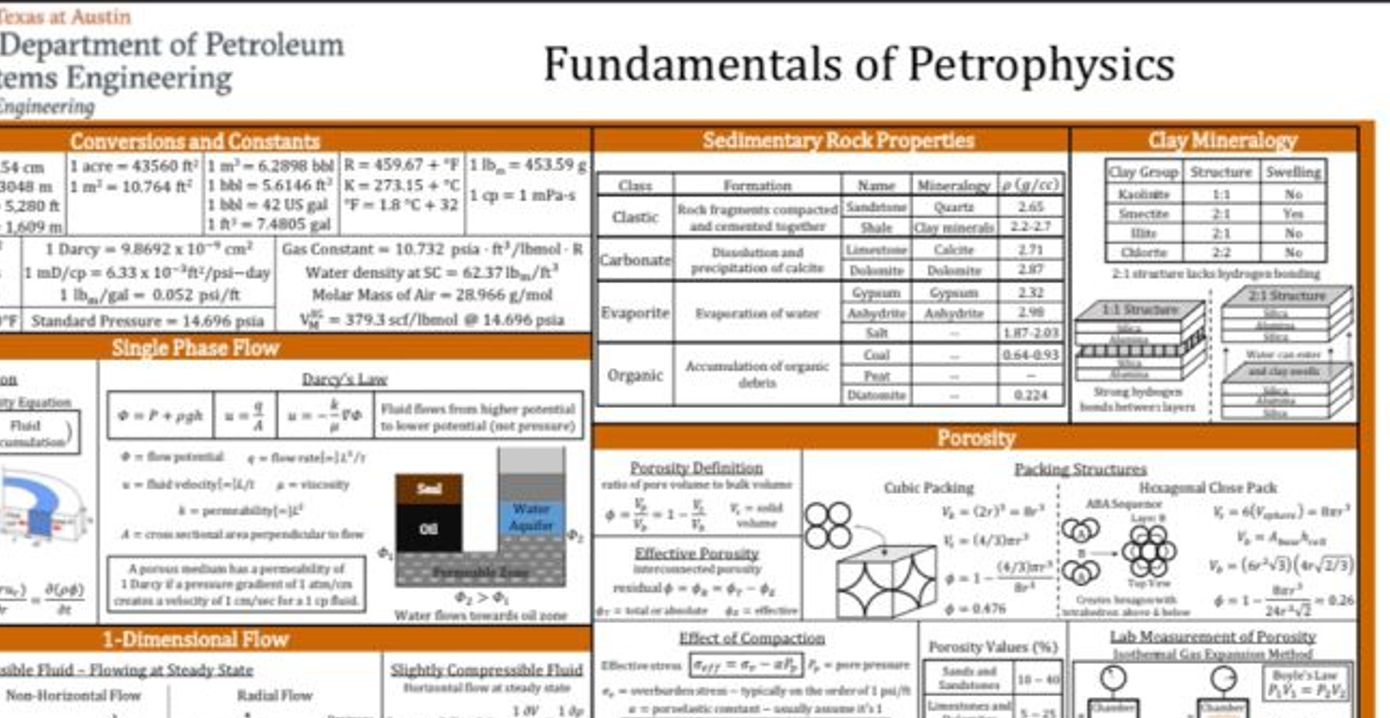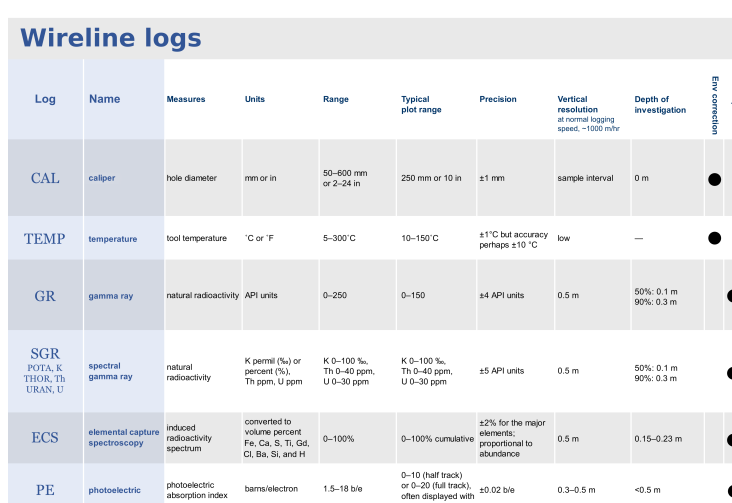## Resources for YOU# QUANTIFYING UNCERTAINTY

Although most petrophysicists do not yet quantify uncertainty, The  Petrophysicist does so routinely using Monte-Carlo simulation and core data (where available). Since software packages are now on the market that allow Monte-Carlo simulation of petrophysical properties to be carried out, our Principal Petrophysicist has written this brief to educate readers on some of the pitfalls with such modelling and how uncertainty is best quantified.

What Is Monte-Carlo Simulation?
When a petrophysical property such as porosity or water saturation is calculated, an interpretation model is assumed. Usually, this model is implemented in a mathematical equation. Petrophysicists typically calculate properties using their most likely estimates of each input required in said equation. In reality though, there is an uncertainty range associated with the inputs used. Uncertainties in the inputs will result in uncertainty in the output, although the magnitude of that uncertainty can be difficult to quantify.

Monte-Carlo simulation provides a simple means by which uncertainties in inputs can be translated into uncertainties in the required petrophysical properties. This simulation is done by selecting a random value from the distribution of likely values for each input parameter required for the interpretation model. Once a value has been selected for all inputs, the interpretation equations are calculated and the result stored. Then the process is repeated and all the results stored. When the requested number of cycles has been completed, the results can be sorted and histograms created allowing the derived petrophysical property at any given probability level to be found.

Meaningful Simulation
It is interesting to note the number of simulations run in some of the advertising for commercially available Petrophysics software incorporating Monte-Carlo simulation. If the number of simulations is small, then the results are unlikely to be meaningful. A minimum number for Monte-carlo simulations to cover a meaningful range of inputs to petrophysical equations is around 1000. Around 10000 simulations is even better, although it does take more time. It is this compromise between time and number of simulations that has limited the use of Monte-Carlo in Petrophysics until computing power has reached a speed such that significant numbers of simulations can be carried out in a reasonable time frame.

Interpretation Model Uncertainty
Since it's not touched upon anywhere else, it MUST be noted that Monte-Carlo simulation only provides uncertainty estimates according to the interpretation model used. It does not address the match between the interpretation model and reality. In practice, this statement means that if your interpretation model is wrong, then uncertainty analysis using Monte-Carlo may not give results that include the "real" answer - which is the objective.

One way to address this problem is to use multiple models to determine the uncertainty. For example, if determining porosity, Monte-Carlo could be run using the density, density-neuton and sonic porosities, in the absence of core data to asist in determining which model is best.

Routine & Special Core Analyses
A better way to quantify the uncertainty is to use the core data if there is any available. The differences between the log and core derived porosities can be used to determine the porosity uncertainty. With water saturations, capillary pressure data (appropriately corrected) can be used to compare with the wireline log derived water saturations to determine uncertainty.

The Recommended Methodology
Of course the best methodology is to select the most appropriate interpretation model and test the resulting petrophysical properties against any core data. In this case, the uncertainties from the comparison with the core data should be contained within the Monte-carlo derived uncertainty distributions. If not, there may be a systematic problem with either the interpretation model (most likely) or the core measurements (also possible).

In the absence of core data, it's recommended to use an interpretation model that has proven itself reliable in a similar Formation in a nearby well. If no such data exists, then the most reliable porosities are normally derived using the density log corrected for hydrocarbons using the invaded zone resistivity, while water saturations estimated using the Waxman-Smits or Dual Water equations are usually most reliable.

## Porosity in Well Logs, Core, NMR## Fundamentals of Petrophysics (QUICK LOOK POSTER)